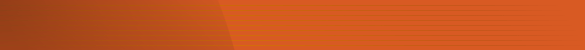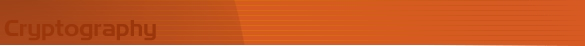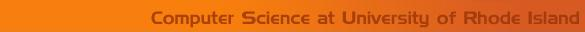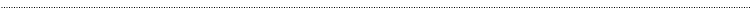Home Page Introduction Classical Ciphers anEnigma Machine DES Key Agreement PGP R.S.A. Number Theory Other LinksClassical CryptographyAffine Cipher

History and Description

Since a shift cipher can produce only 25 different distinct transformations for the text, it is not a very secure encryption method. The affine cipher is a generalization of the shift cipher that provides a little bit more security. The affine cipher applies multiplication and addition to each character using the function:
y = (ax + b) MOD m
where x is the numerical value of the letter in the plaintext, m is the number of letters in the plaintext alphabet, a and b are the secret numbers, and y is the result of transformation. y can be decrypted back to x by using the formula x = inverse(a) (y  b) MOD m, inverse(a) is a value such that if it is multiplied with a MOD m the result will be 1, i.e. (a * inverse(a)) MOD m = 1.

Using the encryption function y = 11x + 4 MOD 26, letter E and S will be encoded to W and U as shown in example below. Since the computation involves modulo 26 arithmetic, several letters may fail to be uniquely decoded if the multiplier  has a common divisor with 26. Therefore, the greatest common divisor of a and m must be 1.

Example

Encipher
Assume the message is encrypted by the function y = (11x + 4) MOD 26
To encrypt the plaintext MONEY, we first convert each letter in plaintext into a numerical value between 0 and 25 according to following list

 A  0 B  1 C  2 D  3 . . . Z - 25

Thus, the numerical values corresponding to the plaintext MONEY are 12, 14, 13, 4, and 24.
Applying the given function for each numerical value, we have

 M: y = (11*12 + 4) MOD 26 = 6 O: y = (11*14 + 4) MOD 26 = 2 N: y = (11*13 + 4) MOD 26 = 17 E : y = (11*4 + 4) MOD 26 = 22 Y : y = (11*24 + 4) MOD 26 = 8

The corresponding letters are GCRWI, which is the ciphertext.

Decipher
To decipher, we transform the function y as:
x = inverse (a) (y  b) MOD m
Then we have, x = inverse(11) (y  4) MOD 26
Inverse(11) MOD 26 = 19, and the decryption function will be x = 19 (y  4) MOD 26

We now decipher the ciphertext GCRWI by applying the decryption function. We have:

 G: x = 19*(6-4) MOD 26 = 12 C: x = 19*(2-4) MOD 26 = 14 R: x = 19*(17-4) MOD 26 = 13 W: x = 19*(22-4) MOD 26 = 4 I: x = 19*(8-4) MOD 26 = 24

The corresponding plaintext letters are MONEY.

Analysis

Since we know that each letter in plaintext is enciphered in the function of y = (ax + b) MOD m, we can break the affine cipher by solving two linear equations with two examples of x and y. Once we obtain the values of a and b, we can decipher the entire  ciphertext.

For example,

Assume that IF is enciphered as PQ.
I -> P: 8a + b = 15 MOD 26
F -> Q: 5a + b = 16 MOD 26
By solving these equations, we have the keys a = 17, b = 9